Скачать презентацию Multimethods Itay Maman Demo Multimethods 1 2 n

927a81301c5b68178573f5834ede9e28.ppt

• Количество слайдов: 43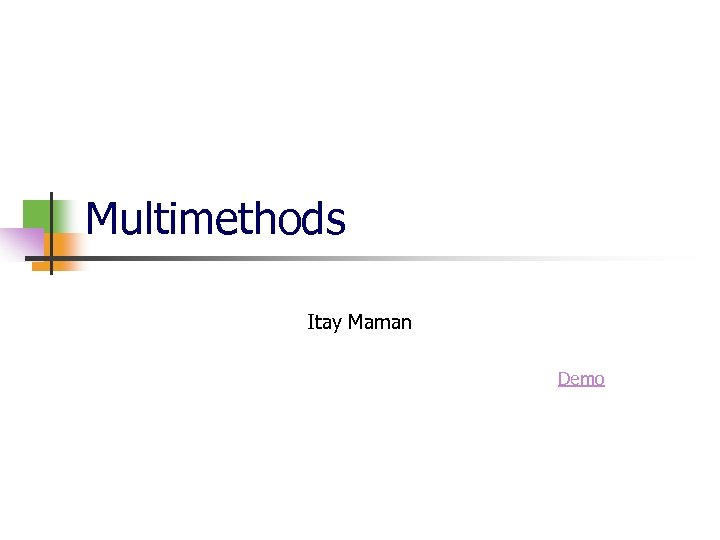Multimethods Itay Maman Demo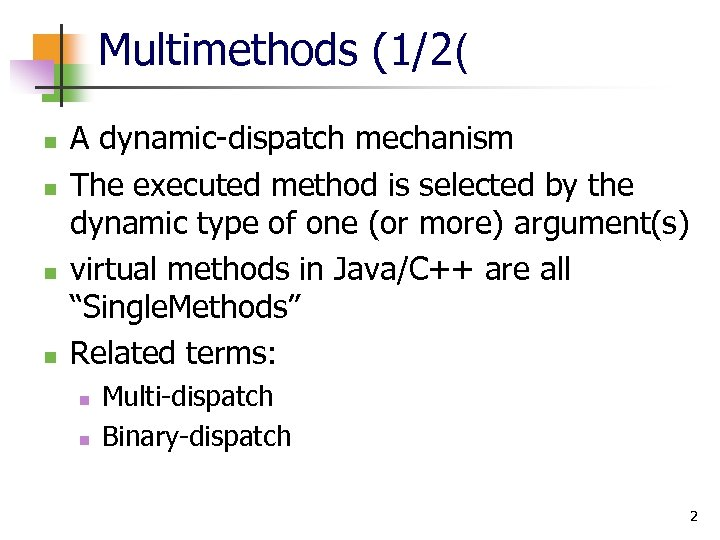Multimethods (1/2( n n A dynamic-dispatch mechanism The executed method is selected by the dynamic type of one (or more) argument(s) virtual methods in Java/C++ are all “Single. Methods” Related terms: n n Multi-dispatch Binary-dispatch 2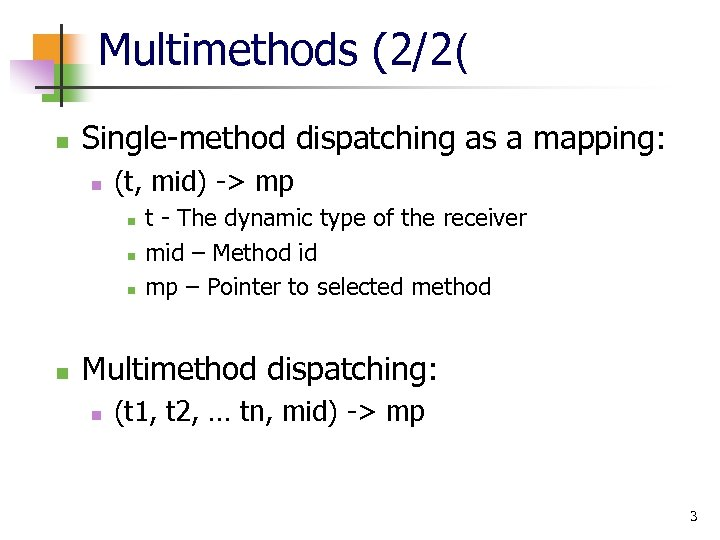Multimethods (2/2( n Single-method dispatching as a mapping: n (t, mid) -> mp n n t - The dynamic type of the receiver mid – Method id mp – Pointer to selected method Multimethod dispatching: n (t 1, t 2, … tn, mid) -> mp 3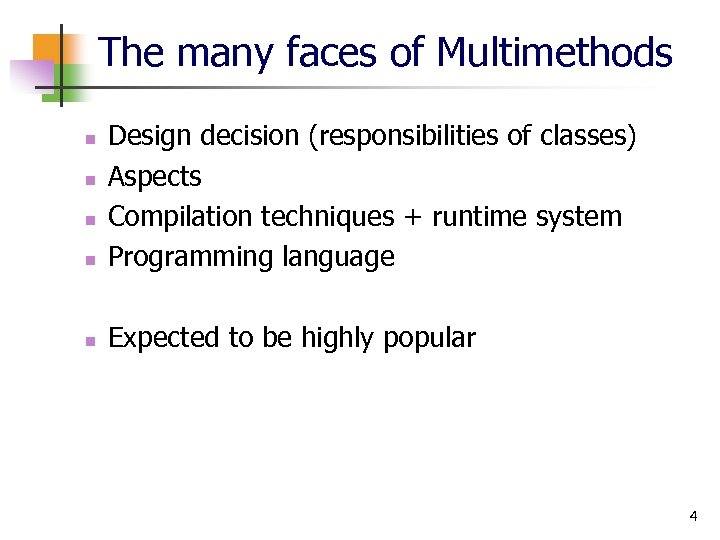The many faces of Multimethods n Design decision (responsibilities of classes) Aspects Compilation techniques + runtime system Programming language n Expected to be highly popular n n n 4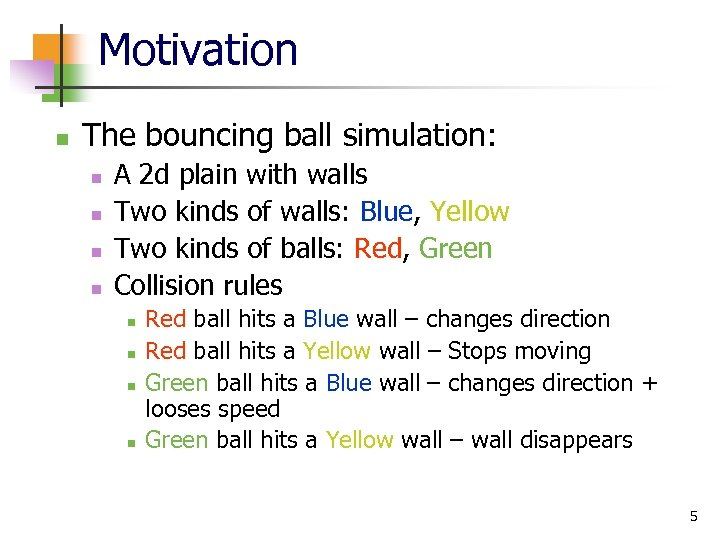Motivation n The bouncing ball simulation: n n A 2 d plain with walls Two kinds of walls: Blue, Yellow Two kinds of balls: Red, Green Collision rules n n Red ball hits a Blue wall – changes direction Red ball hits a Yellow wall – Stops moving Green ball hits a Blue wall – changes direction + looses speed Green ball hits a Yellow wall – wall disappears 5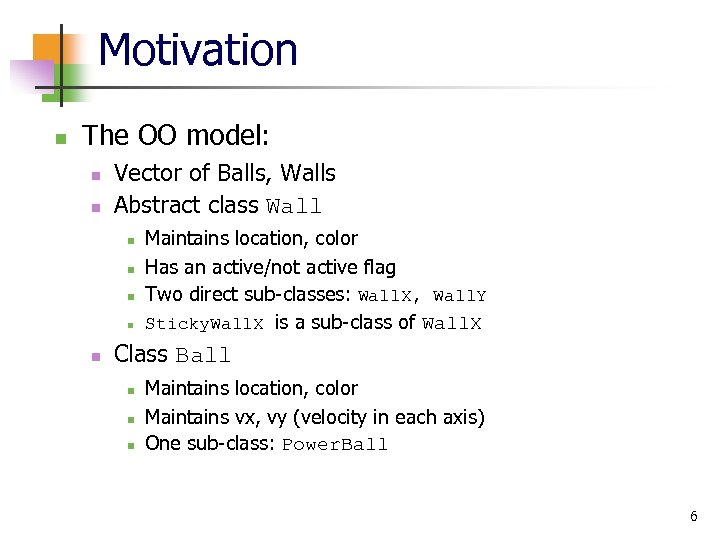Motivation n The OO model: n n Vector of Balls, Walls Abstract class Wall n n n Maintains location, color Has an active/not active flag Two direct sub-classes: Wall. X, Wall. Y Sticky. Wall. X is a sub-class of Wall. X Class Ball n n n Maintains location, color Maintains vx, vy (velocity in each axis) One sub-class: Power. Ball 6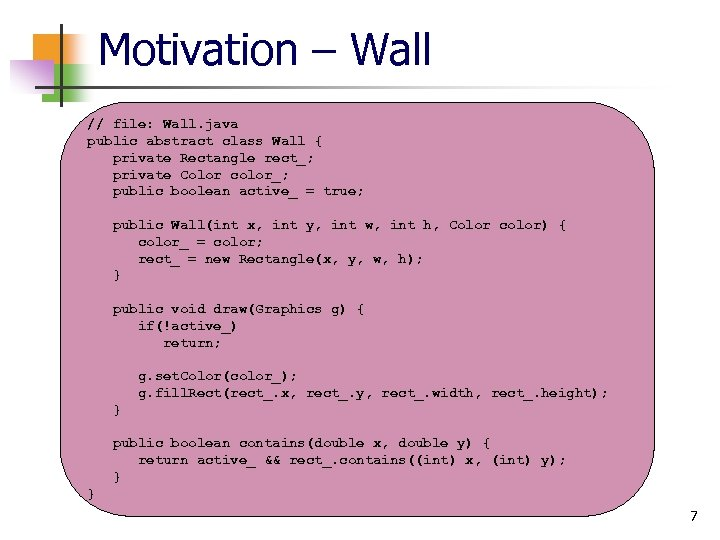Motivation – Wall // file: Wall. java public abstract class Wall { private Rectangle rect_; private Color color_; public boolean active_ = true; public Wall(int x, int y, int w, int h, Color color) { color_ = color; rect_ = new Rectangle(x, y, w, h); } public void draw(Graphics g) { if(!active_) return; g. set. Color(color_); g. fill. Rect(rect_. x, rect_. y, rect_. width, rect_. height); } public boolean contains(double x, double y) { return active_ && rect_. contains((int) x, (int) y); } } 7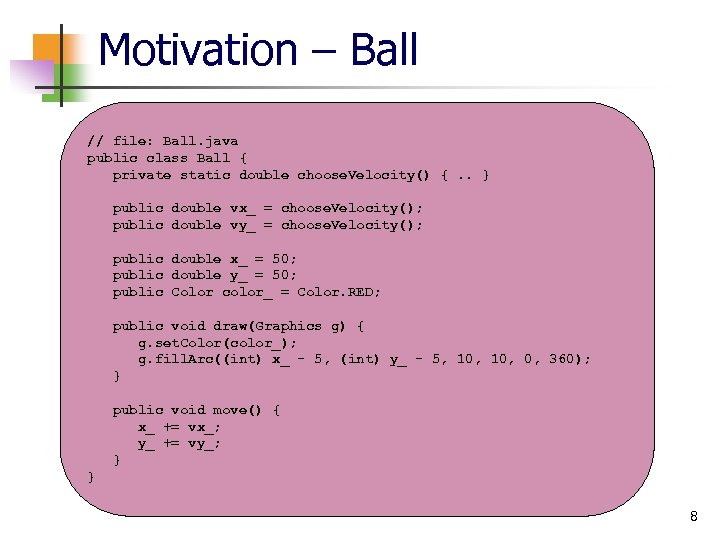Motivation – Ball // file: Ball. java public class Ball { private static double choose. Velocity() {. . } public double vx_ = choose. Velocity(); public double vy_ = choose. Velocity(); public double x_ = 50; public double y_ = 50; public Color color_ = Color. RED; public void draw(Graphics g) { g. set. Color(color_); g. fill. Arc((int) x_ - 5, (int) y_ - 5, 10, 0, 360); } public void move() { x_ += vx_; y_ += vy_; } } 8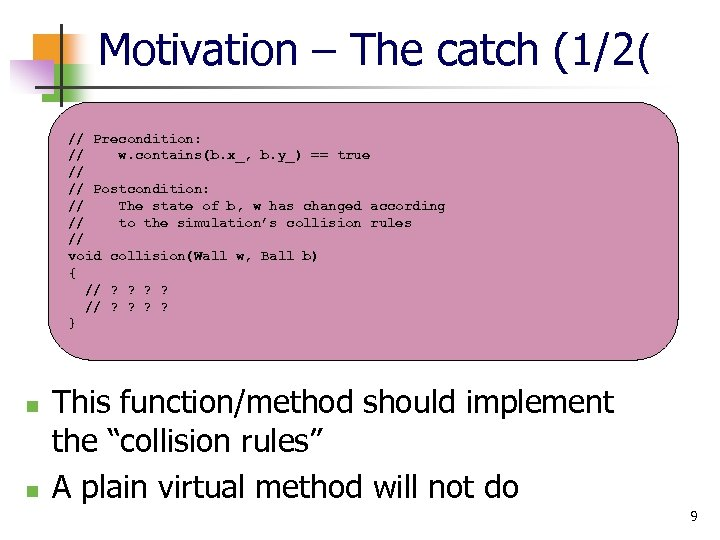Motivation – The catch (1/2( // Precondition: // w. contains(b. x_, b. y_) == true // // Postcondition: // The state of b, w has changed according // to the simulation’s collision rules // void collision(Wall w, Ball b) { // ? ? ? ? } n n This function/method should implement the “collision rules” A plain virtual method will not do 9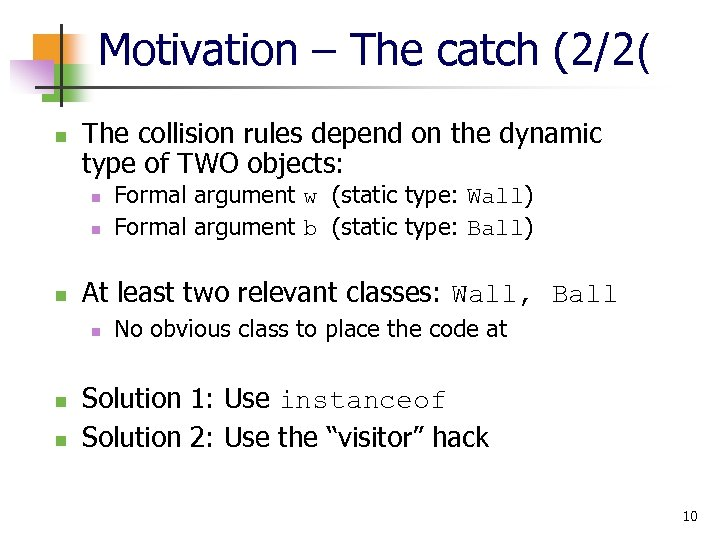Motivation – The catch (2/2( n The collision rules depend on the dynamic type of TWO objects: n n n At least two relevant classes: Wall, Ball n n n Formal argument w (static type: Wall) Formal argument b (static type: Ball) No obvious class to place the code at Solution 1: Use instanceof Solution 2: Use the “visitor” hack 10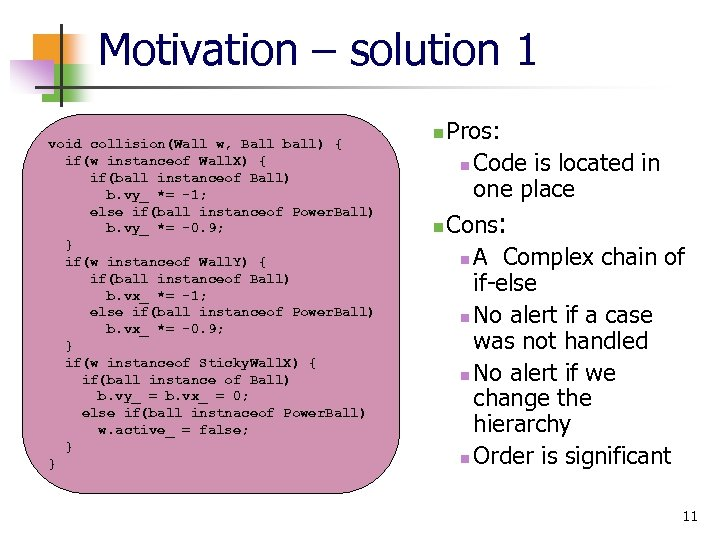Motivation – solution 1 void collision(Wall w, Ball ball) { if(w instanceof Wall. X) { if(ball instanceof Ball) b. vy_ *= -1; else if(ball instanceof Power. Ball) b. vy_ *= -0. 9; } if(w instanceof Wall. Y) { if(ball instanceof Ball) b. vx_ *= -1; else if(ball instanceof Power. Ball) b. vx_ *= -0. 9; } if(w instanceof Sticky. Wall. X) { if(ball instance of Ball) b. vy_ = b. vx_ = 0; else if(ball instnaceof Power. Ball) w. active_ = false; } } n n Pros: n Code is located in one place Cons: n A Complex chain of if-else n No alert if a case was not handled n No alert if we change the hierarchy n Order is significant 11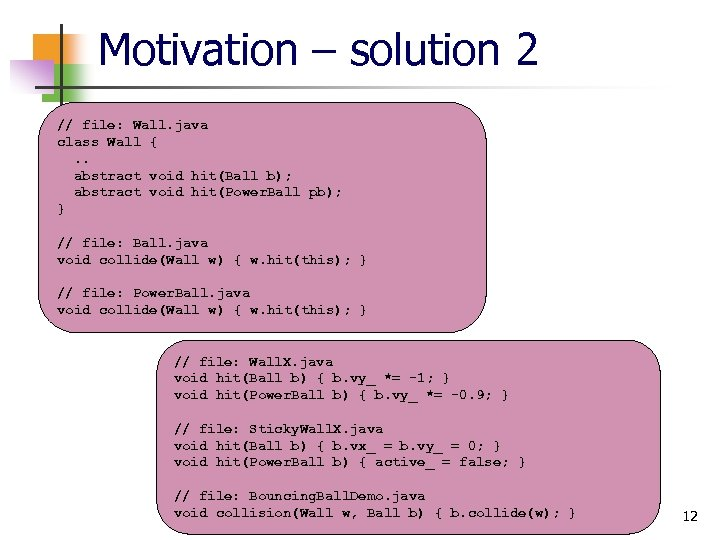Motivation – solution 2 // file: Wall. java class Wall {. . abstract void hit(Ball b); abstract void hit(Power. Ball pb); } // file: Ball. java void collide(Wall w) { w. hit(this); } // file: Power. Ball. java void collide(Wall w) { w. hit(this); } // file: Wall. X. java void hit(Ball b) { b. vy_ *= -1; } void hit(Power. Ball b) { b. vy_ *= -0. 9; } // file: Sticky. Wall. X. java void hit(Ball b) { b. vx_ = b. vy_ = 0; } void hit(Power. Ball b) { active_ = false; } // file: Bouncing. Ball. Demo. java void collision(Wall w, Ball b) { b. collide(w); } 12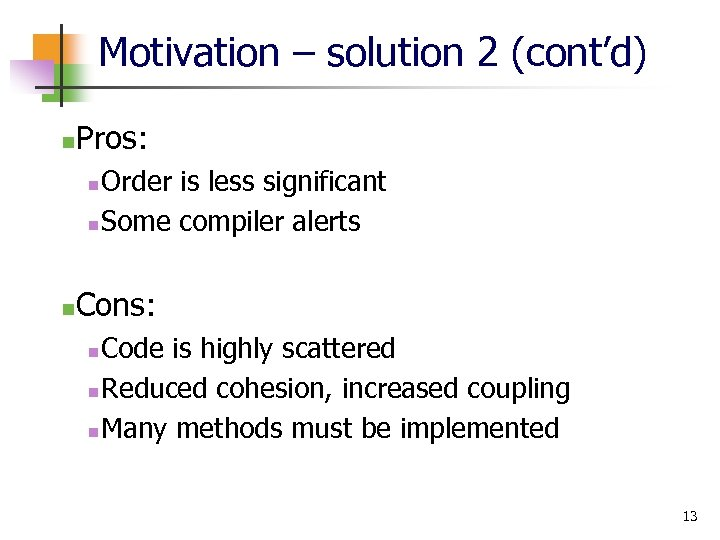Motivation – solution 2 (cont’d) n Pros: Order is less significant n Some compiler alerts n n Cons: Code is highly scattered n Reduced cohesion, increased coupling n Many methods must be implemented n 13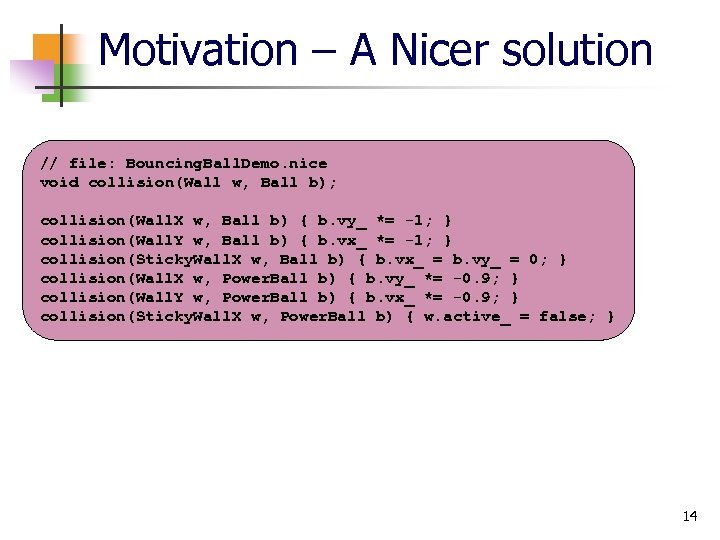Motivation – A Nicer solution // file: Bouncing. Ball. Demo. nice void collision(Wall w, Ball b); collision(Wall. X w, Ball b) { b. vy_ *= -1; } collision(Wall. Y w, Ball b) { b. vx_ *= -1; } collision(Sticky. Wall. X w, Ball b) { b. vx_ = b. vy_ = 0; } collision(Wall. X w, Power. Ball b) { b. vy_ *= -0. 9; } collision(Wall. Y w, Power. Ball b) { b. vx_ *= -0. 9; } collision(Sticky. Wall. X w, Power. Ball b) { w. active_ = false; } 14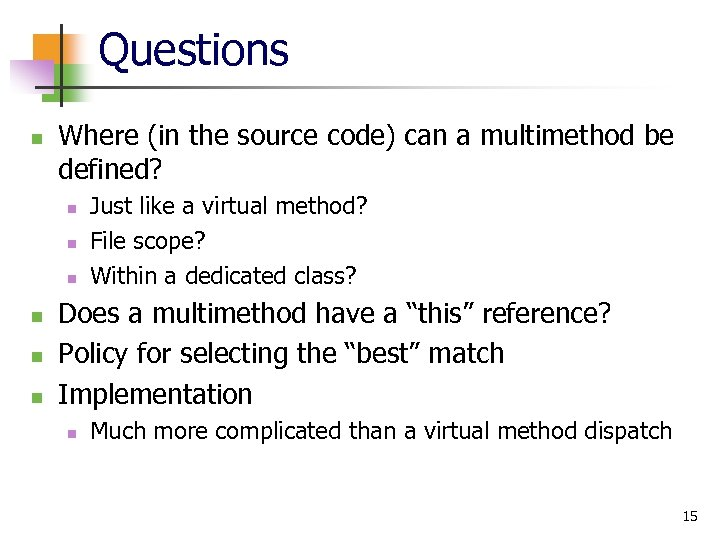Questions n Where (in the source code) can a multimethod be defined? n n n Just like a virtual method? File scope? Within a dedicated class? Does a multimethod have a “this” reference? Policy for selecting the “best” match Implementation n Much more complicated than a virtual method dispatch 15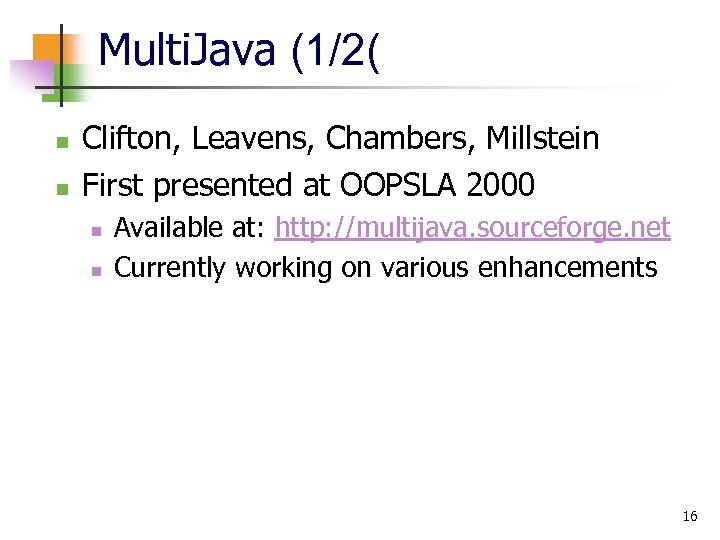Multi. Java (1/2( n n Clifton, Leavens, Chambers, Millstein First presented at OOPSLA 2000 n n Available at: http: //multijava. sourceforge. net Currently working on various enhancements 16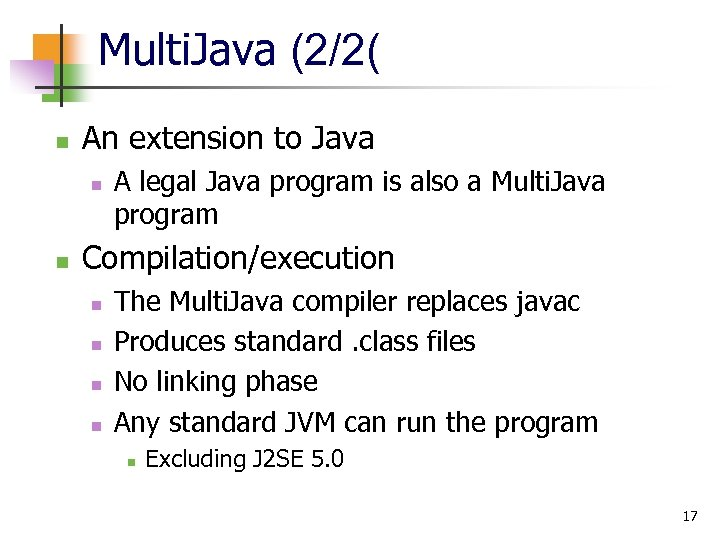Multi. Java (2/2( n An extension to Java n n A legal Java program is also a Multi. Java program Compilation/execution n n The Multi. Java compiler replaces javac Produces standard. class files No linking phase Any standard JVM can run the program n Excluding J 2 SE 5. 0 17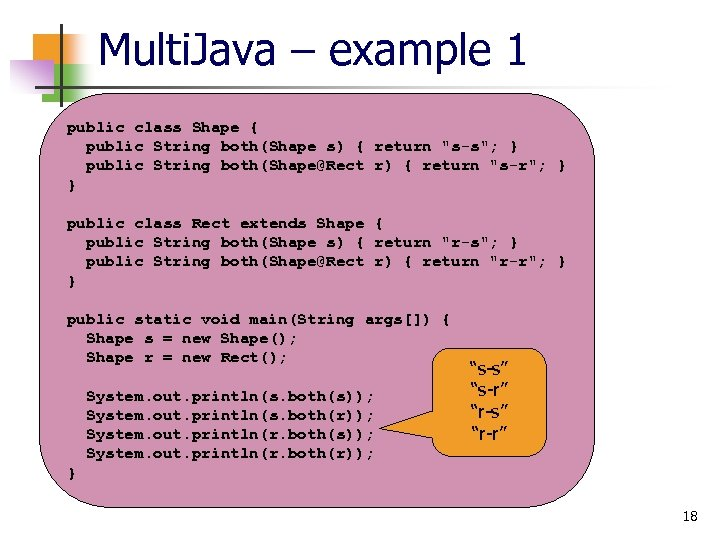Multi. Java – example 1 public class Shape { public String both(Shape s) { return "s-s"; } public String both([email protected] r) { return "s-r"; } } public class Rect extends Shape { public String both(Shape s) { return "r-s"; } public String both([email protected] r) { return "r-r"; } } public static void main(String args[]) { Shape s = new Shape(); Shape r = new Rect(); System. out. println(s. both(s)); System. out. println(s. both(r)); System. out. println(r. both(s)); System. out. println(r. both(r)); “s-s” “s-r” “r-s” “r-r” } 18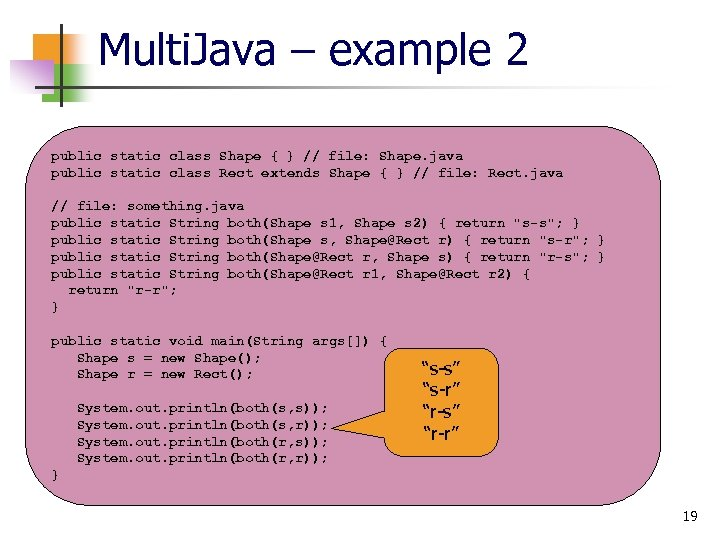Multi. Java – example 2 public static class Shape { } // file: Shape. java public static class Rect extends Shape { } // file: Rect. java // file: something. java public static String both(Shape s 1, Shape s 2) { return "s-s"; } public static String both(Shape s, [email protected] r) { return "s-r"; } public static String both([email protected] r, Shape s) { return "r-s"; } public static String both([email protected] r 1, [email protected] r 2) { return "r-r"; } public static void main(String args[]) { Shape s = new Shape(); Shape r = new Rect(); System. out. println(both(s, s)); System. out. println(both(s, r)); System. out. println(both(r, s)); System. out. println(both(r, r)); “s-s” “s-r” “r-s” “r-r” } 19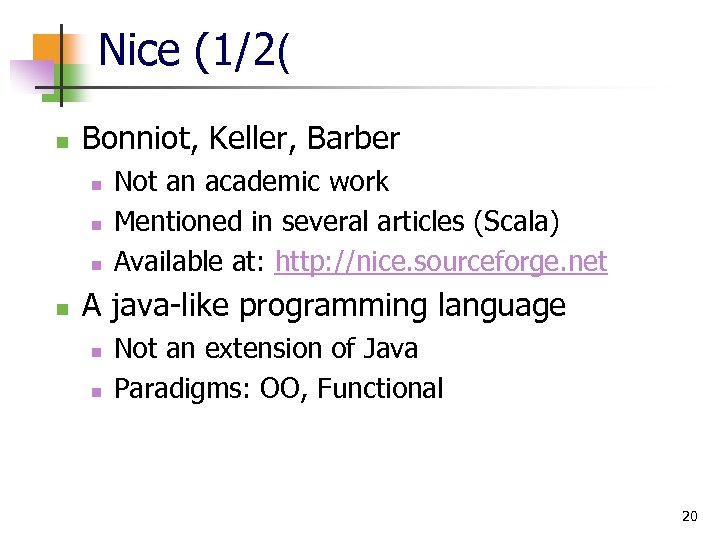Nice (1/2( n Bonniot, Keller, Barber n n Not an academic work Mentioned in several articles (Scala) Available at: http: //nice. sourceforge. net A java-like programming language n n Not an extension of Java Paradigms: OO, Functional 20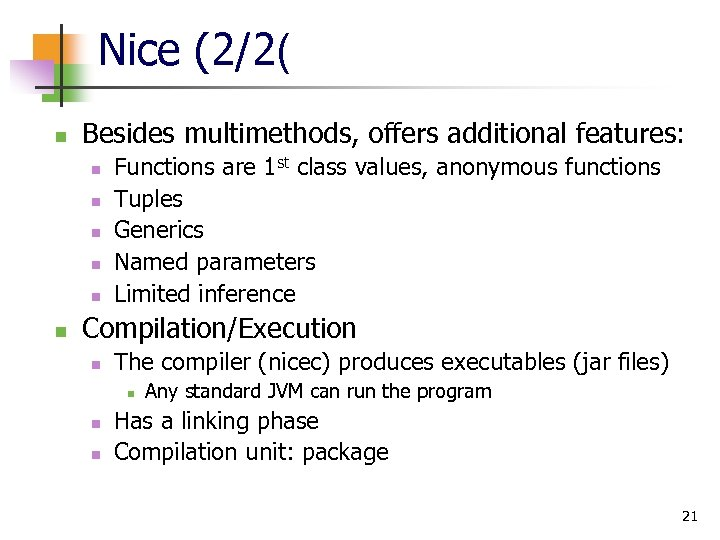Nice (2/2( n Besides multimethods, offers additional features: n n n Functions are 1 st class values, anonymous functions Tuples Generics Named parameters Limited inference Compilation/Execution n The compiler (nicec) produces executables (jar files) n n n Any standard JVM can run the program Has a linking phase Compilation unit: package 21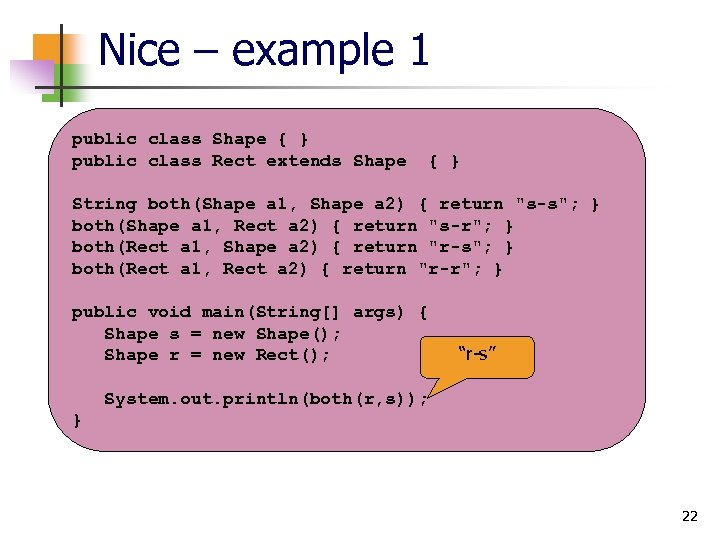Nice – example 1 public class Shape { } public class Rect extends Shape { } String both(Shape a 1, Shape a 2) { return "s-s"; } both(Shape a 1, Rect a 2) { return "s-r"; } both(Rect a 1, Shape a 2) { return "r-s"; } both(Rect a 1, Rect a 2) { return "r-r"; } public void main(String[] args) { Shape s = new Shape(); Shape r = new Rect(); “r-s” System. out. println(both(r, s)); } 22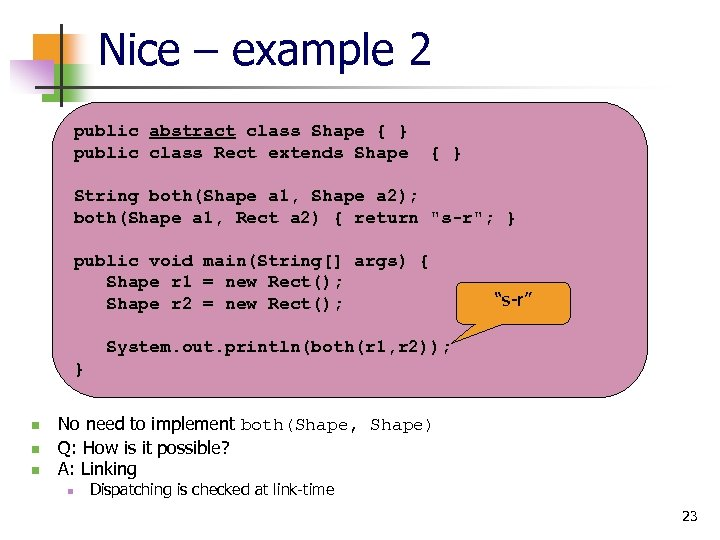Nice – example 2 public abstract class Shape { } public class Rect extends Shape { } String both(Shape a 1, Shape a 2); both(Shape a 1, Rect a 2) { return "s-r"; } public void main(String[] args) { Shape r 1 = new Rect(); Shape r 2 = new Rect(); “s-r” System. out. println(both(r 1, r 2)); } n n n No need to implement both(Shape, Shape) Q: How is it possible? A: Linking n Dispatching is checked at link-time 23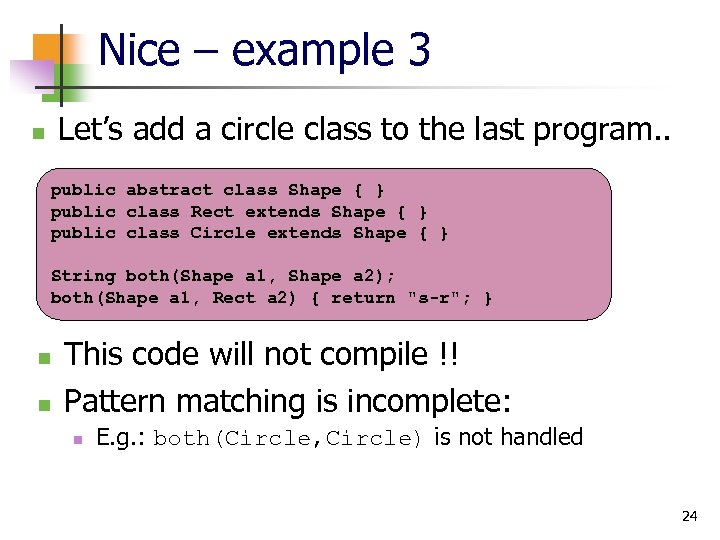Nice – example 3 Let’s add a circle class to the last program. . n public abstract class Shape { } public class Rect extends Shape { } public class Circle extends Shape { } String both(Shape a 1, Shape a 2); both(Shape a 1, Rect a 2) { return "s-r"; } n n This code will not compile !! Pattern matching is incomplete: n E. g. : both(Circle, Circle) is not handled 24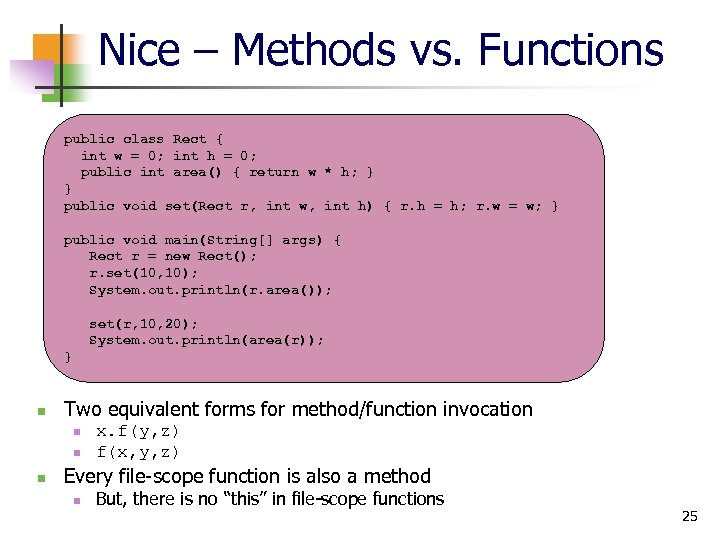Nice – Methods vs. Functions public class Rect { int w = 0; int h = 0; public int area() { return w * h; } } public void set(Rect r, int w, int h) { r. h = h; r. w = w; } public void main(String[] args) { Rect r = new Rect(); r. set(10, 10); System. out. println(r. area()); set(r, 10, 20); System. out. println(area(r)); } n Two equivalent forms for method/function invocation n x. f(y, z) f(x, y, z) Every file-scope function is also a method n But, there is no “this” in file-scope functions 25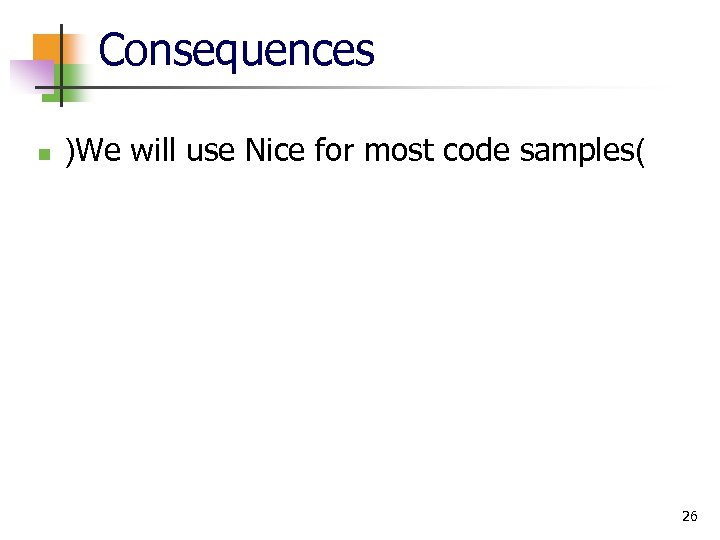Consequences n )We will use Nice for most code samples( 26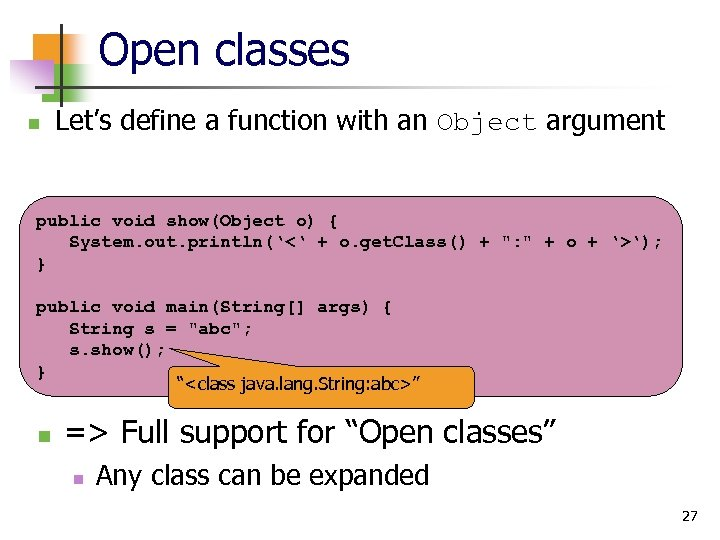Open classes n Let’s define a function with an Object argument public void show(Object o) { System. out. println(‘<‘ + o. get. Class() + ": " + o + ‘>‘); } public void main(String[] args) { String s = "abc"; s. show(); } “” n => Full support for “Open classes” n Any class can be expanded 27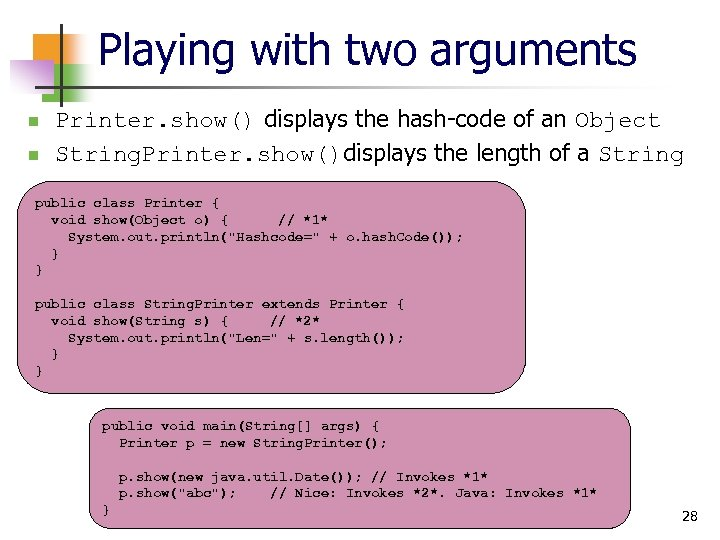Playing with two arguments n n Printer. show() displays the hash-code of an Object String. Printer. show()displays the length of a String public class Printer { void show(Object o) { // *1* System. out. println("Hashcode=" + o. hash. Code()); } } public class String. Printer extends Printer { void show(String s) { // *2* System. out. println("Len=" + s. length()); } } public void main(String[] args) { Printer p = new String. Printer(); p. show(new java. util. Date()); // Invokes *1* p. show("abc"); // Nice: Invokes *2*. Java: Invokes *1* } 28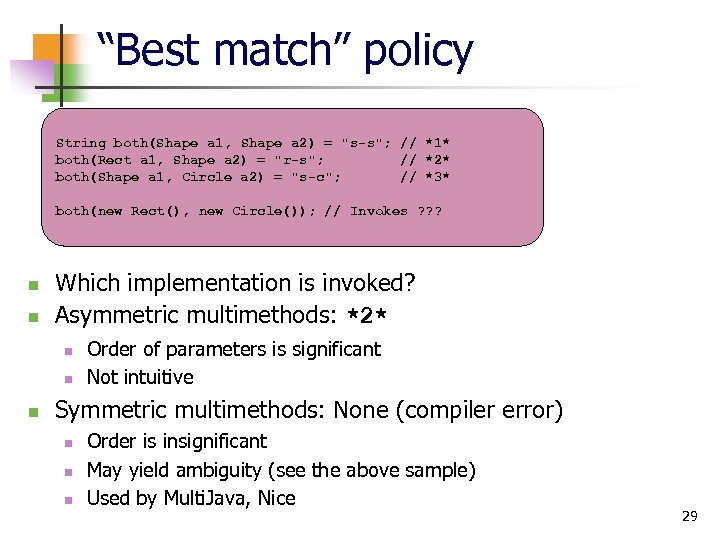“Best match” policy String both(Shape a 1, Shape a 2) = "s-s"; // *1* both(Rect a 1, Shape a 2) = "r-s"; // *2* both(Shape a 1, Circle a 2) = "s-c"; // *3* both(new Rect(), new Circle()); // Invokes ? ? ? n n Which implementation is invoked? Asymmetric multimethods: *2* n n n Order of parameters is significant Not intuitive Symmetric multimethods: None (compiler error) n n n Order is insignificant May yield ambiguity (see the above sample) Used by Multi. Java, Nice 29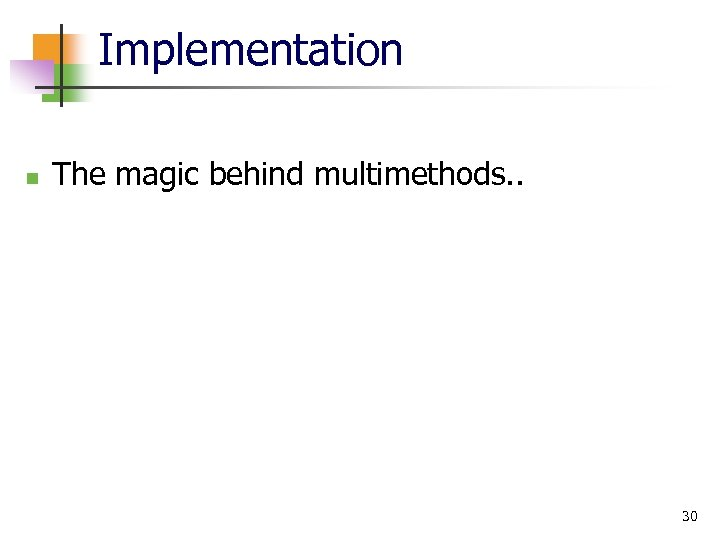Implementation n The magic behind multimethods. . 30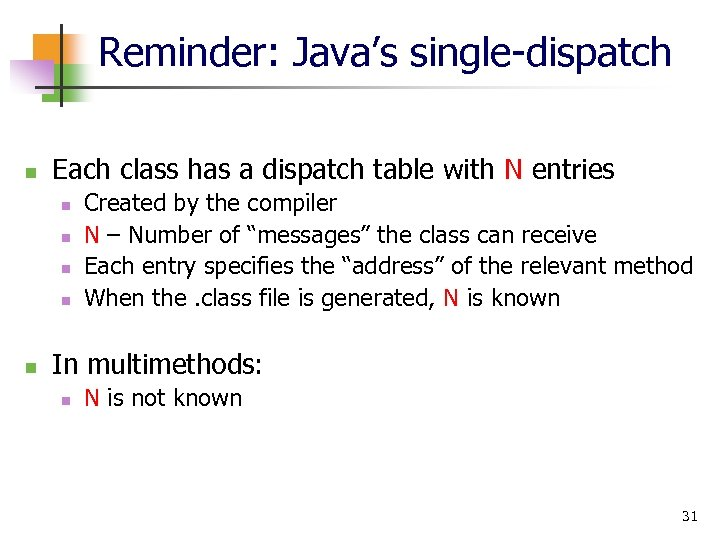Reminder: Java’s single-dispatch n Each class has a dispatch table with N entries n n n Created by the compiler N – Number of “messages” the class can receive Each entry specifies the “address” of the relevant method When the. class file is generated, N is known In multimethods: n N is not known 31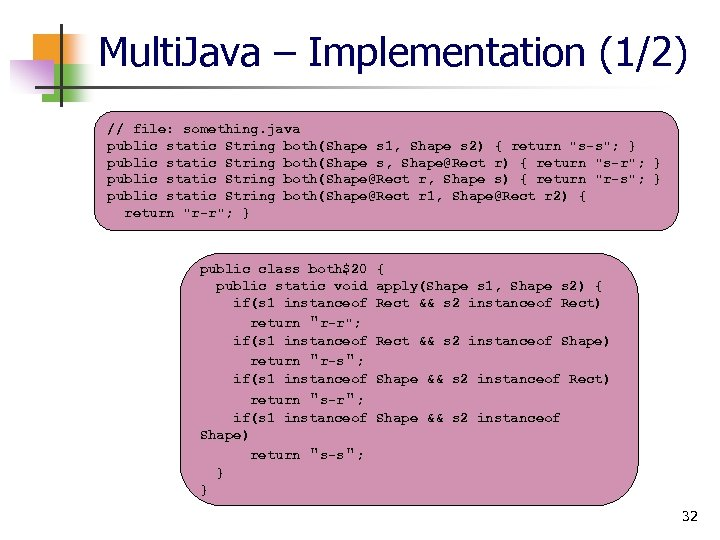Multi. Java – Implementation (1/2) // file: something. java public static String both(Shape s 1, Shape s 2) { return "s-s"; } public static String both(Shape s, [email protected] r) { return "s-r"; } public static String both([email protected] r, Shape s) { return "r-s"; } public static String both([email protected] r 1, [email protected] r 2) { return "r-r"; } public class both\$20 public static void if(s 1 instanceof return "r-r"; if(s 1 instanceof return "r-s"; if(s 1 instanceof return "s-r"; if(s 1 instanceof Shape) return "s-s"; } } { apply(Shape s 1, Shape s 2) { Rect && s 2 instanceof Rect) Rect && s 2 instanceof Shape) Shape && s 2 instanceof Rect) Shape && s 2 instanceof 32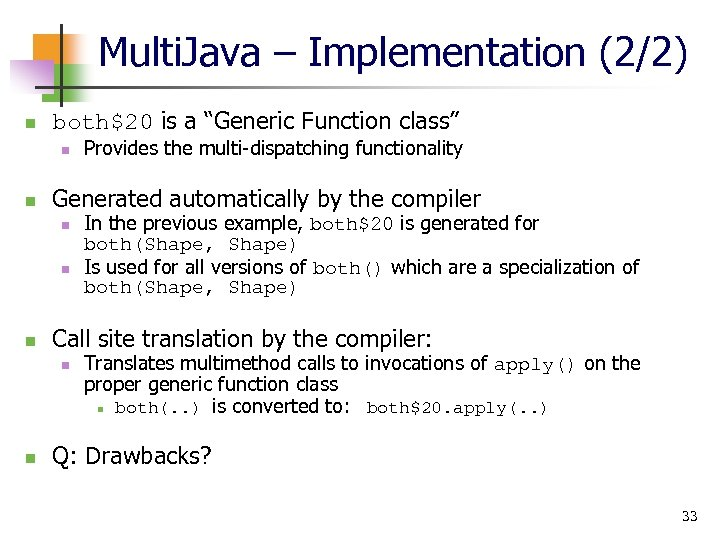Multi. Java – Implementation (2/2) n both\$20 is a “Generic Function class” n n Generated automatically by the compiler n n n In the previous example, both\$20 is generated for both(Shape, Shape) Is used for all versions of both() which are a specialization of both(Shape, Shape) Call site translation by the compiler: n n Provides the multi-dispatching functionality Translates multimethod calls to invocations of apply() on the proper generic function class n both(. . ) is converted to: both\$20. apply(. . ) Q: Drawbacks? 33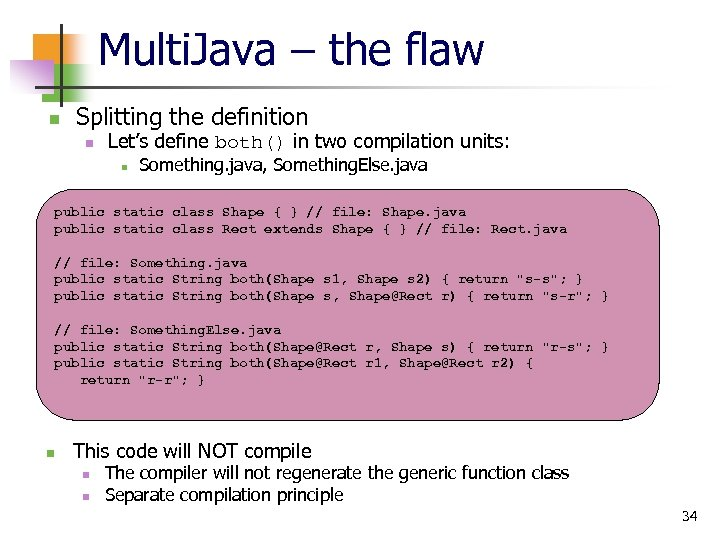Multi. Java – the flaw n Splitting the definition n Let’s define both() in two compilation units: n Something. java, Something. Else. java public static class Shape { } // file: Shape. java public static class Rect extends Shape { } // file: Rect. java // file: Something. java public static String both(Shape s 1, Shape s 2) { return "s-s"; } public static String both(Shape s, [email protected] r) { return "s-r"; } // file: Something. Else. java public static String both([email protected] r, Shape s) { return "r-s"; } public static String both([email protected] r 1, [email protected] r 2) { return "r-r"; } n This code will NOT compile n n The compiler will not regenerate the generic function class Separate compilation principle 34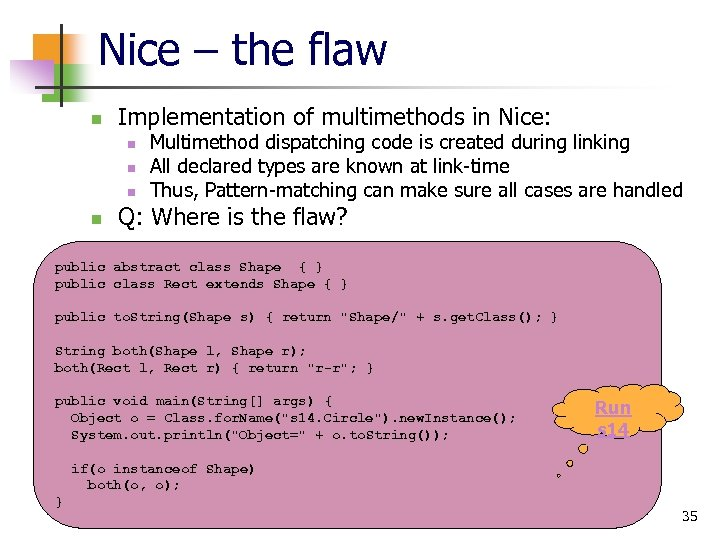Nice – the flaw n Implementation of multimethods in Nice: n n Multimethod dispatching code is created during linking All declared types are known at link-time Thus, Pattern-matching can make sure all cases are handled Q: Where is the flaw? public abstract class Shape { } public class Rect extends Shape { } public to. String(Shape s) { return "Shape/" + s. get. Class(); } String both(Shape l, Shape r); both(Rect l, Rect r) { return "r-r"; } public void main(String[] args) { Object o = Class. for. Name("s 14. Circle"). new. Instance(); System. out. println("Object=" + o. to. String()); Run s 14 if(o instanceof Shape) both(o, o); } 35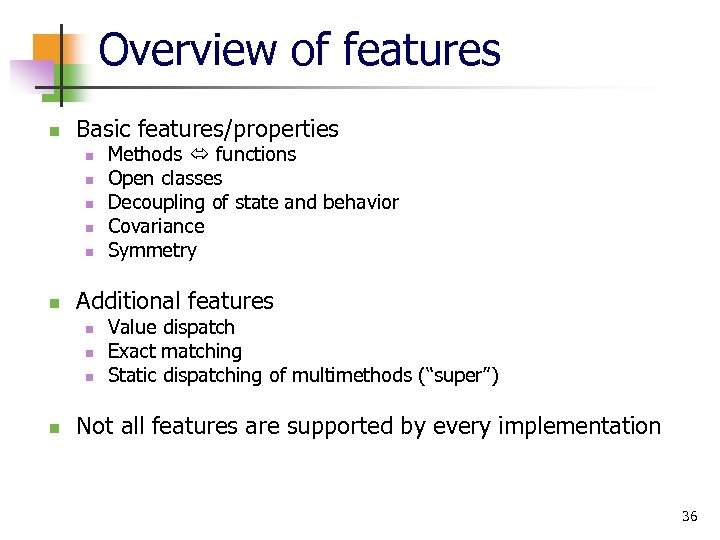Overview of features n Basic features/properties n n n Additional features n n Methods functions Open classes Decoupling of state and behavior Covariance Symmetry Value dispatch Exact matching Static dispatching of multimethods (“super”) Not all features are supported by every implementation 36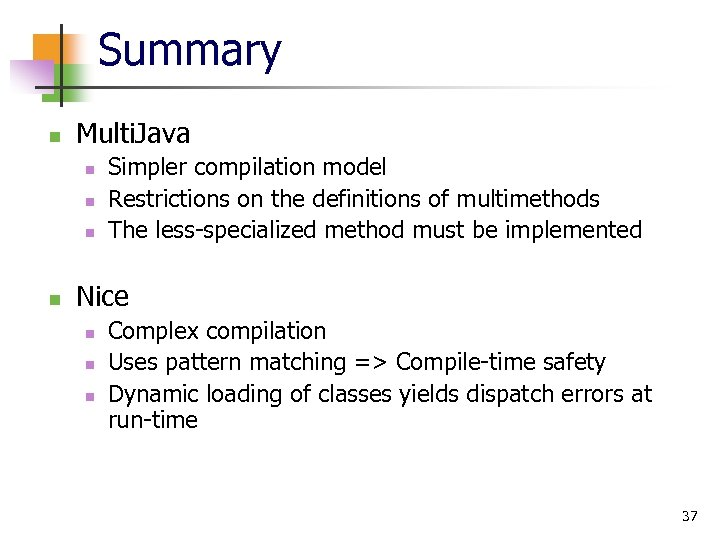Summary n Multi. Java n n Simpler compilation model Restrictions on the definitions of multimethods The less-specialized method must be implemented Nice n n n Complex compilation Uses pattern matching => Compile-time safety Dynamic loading of classes yields dispatch errors at run-time 37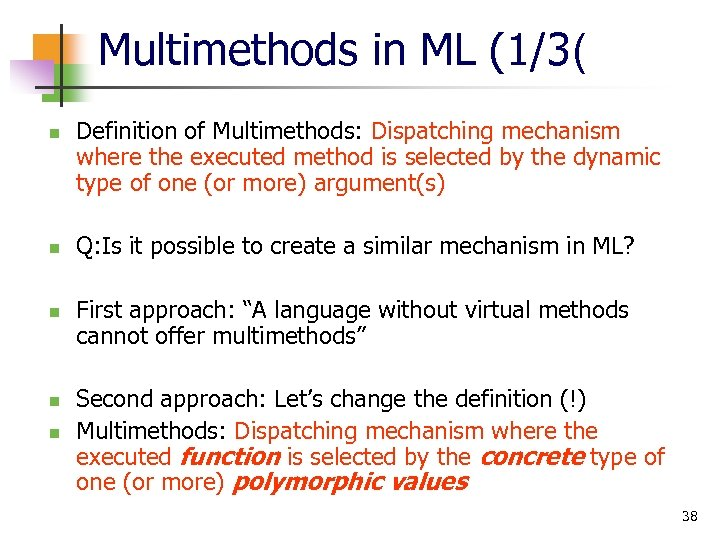Multimethods in ML (1/3( n n n Definition of Multimethods: Dispatching mechanism where the executed method is selected by the dynamic type of one (or more) argument(s) Q: Is it possible to create a similar mechanism in ML? First approach: “A language without virtual methods cannot offer multimethods” Second approach: Let’s change the definition (!) Multimethods: Dispatching mechanism where the executed function is selected by the concrete type of one (or more) polymorphic values 38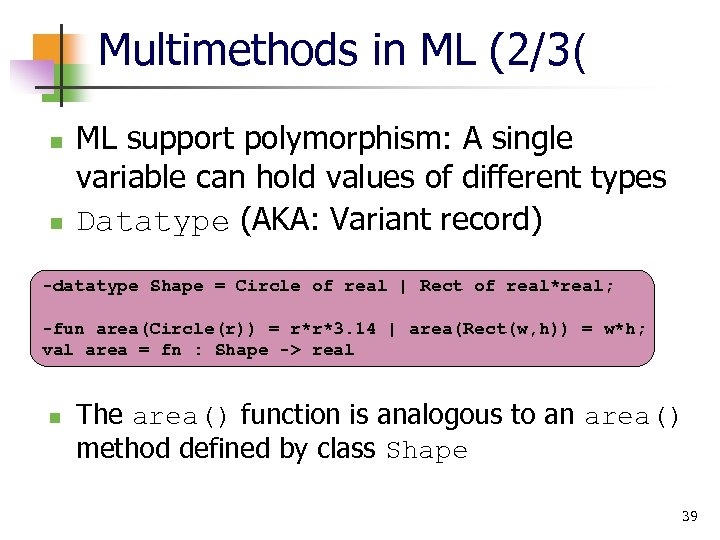Multimethods in ML (2/3( n n ML support polymorphism: A single variable can hold values of different types Datatype (AKA: Variant record) -datatype Shape = Circle of real | Rect of real*real; -fun area(Circle(r)) = r*r*3. 14 | area(Rect(w, h)) = w*h; val area = fn : Shape -> real n The area() function is analogous to an area() method defined by class Shape 39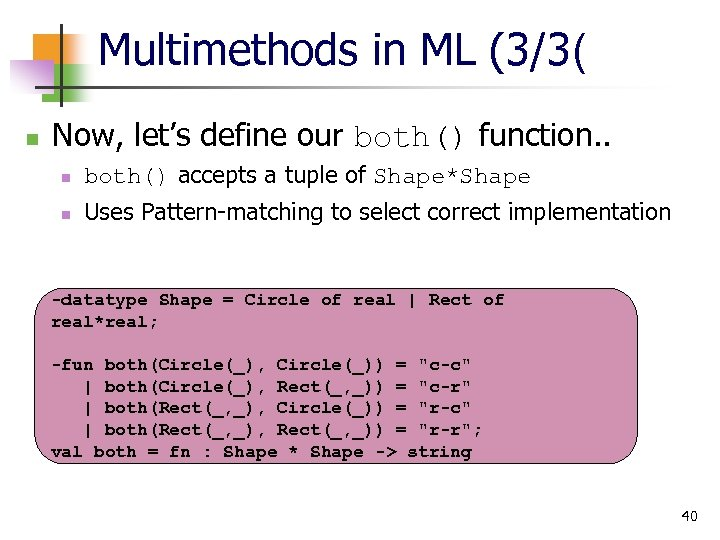Multimethods in ML (3/3( n Now, let’s define our both() function. . n both() accepts a tuple of Shape*Shape n Uses Pattern-matching to select correct implementation -datatype Shape = Circle of real | Rect of real*real; -fun both(Circle(_), Circle(_)) = "c-c" | both(Circle(_), Rect(_, _)) = "c-r" | both(Rect(_, _), Circle(_)) = "r-c" | both(Rect(_, _), Rect(_, _)) = "r-r"; val both = fn : Shape * Shape -> string 40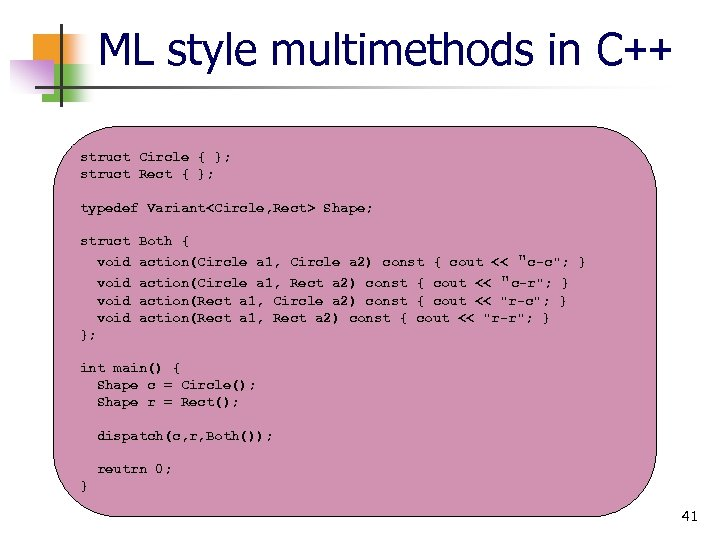ML style multimethods in C++ struct Circle { }; struct Rect { }; typedef Variant Shape; struct Both { void action(Circle a 1, Circle a 2) const { cout << "c-c"; } void action(Circle a 1, Rect a 2) const { cout << "c-r"; } void action(Rect a 1, Circle a 2) const { cout << "r-c"; } void action(Rect a 1, Rect a 2) const { cout << "r-r"; } }; int main() { Shape c = Circle(); Shape r = Rect(); dispatch(c, r, Both()); reutrn 0; } 41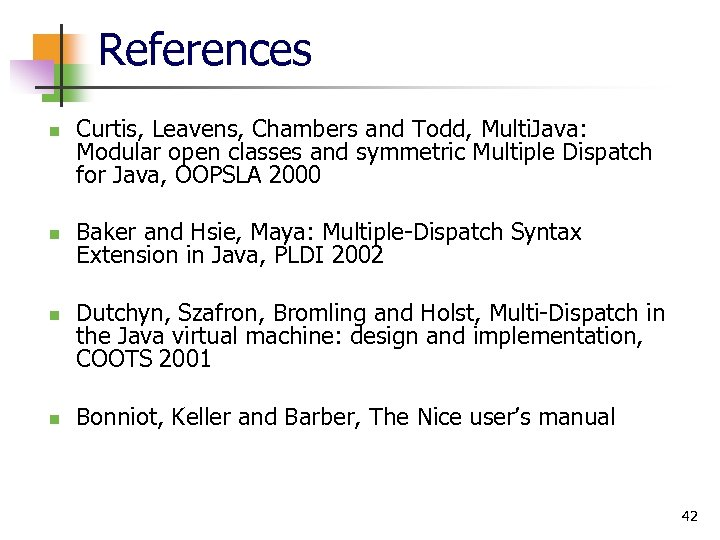References n n Curtis, Leavens, Chambers and Todd, Multi. Java: Modular open classes and symmetric Multiple Dispatch for Java, OOPSLA 2000 Baker and Hsie, Maya: Multiple-Dispatch Syntax Extension in Java, PLDI 2002 Dutchyn, Szafron, Bromling and Holst, Multi-Dispatch in the Java virtual machine: design and implementation, COOTS 2001 Bonniot, Keller and Barber, The Nice user’s manual 42-The End- 43